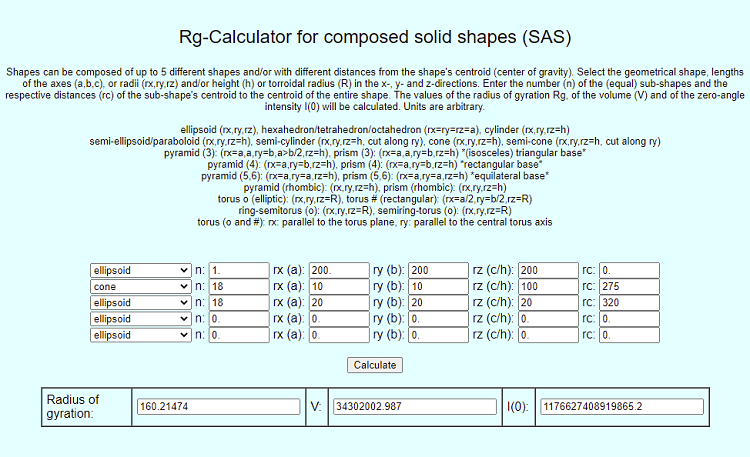Cone-spiked sphere ressembling a simplified Coronavirus shape

Select with the sliders in the upper left corner the shape of the spikes (cones: slider to the left, inverted cones: slider to the right, cylinders: slider in the middle), radius of the cones or cylinders (rc) and their length (rl) and the radius of the center sphere (rr) as well of the spheres attached to the end of the spikes (rs), the speed of 3D-rotation of the model (speed) and the greyscale of the background (back). The cones or cylinders (18 distributed symmetrically) are touching the surface of the central sphere. The distance from the center of gravity of the entire model to the center of gravity of the surrounding spikes is equal to rr + x*rl (with x being 0.25 for cones, 0.75 for inverted cones and 0.5 for cylinders, respectively), and to the center of the spike-spheres is equal to rr + rl + rs. The resulting volume (V) and the Radius of Gyration (RG) for the model with the selected parameters are displayed (assuming uniform and constant electron density or scattering length density) having the same (or related) units as the input parameters. A similar Javascript-animated model can be found here.
A detailed nanostructural model of the Coronavirus is illustrated here (static) or here (animated). Realistic nanostructural size dimensions are: total diameter ~ 100 nm, length of the (approximately 100 per virion) spike-proteins (trimers) ~ 10 nm. Calculating the RG and V for the default model (rr=200, rc=10, rl=100, rs=20 and with the resulting distance of the center of gravity of the 18 inverted cones to the center of the sphere being 200 + 3/4*100 = 275, and of the 18 spike-spheres to the center being 200 + 100 + 20 = 320) these parameters are entered as input in the Rg-Calculator for composed solid shapes as shown below.Author: M.Kriechbaum, TU-Graz (2021), e-mail: manfred.kriechbaum@tugraz.at